# Balancing Chemical Equations. Balancing redox equationsPage 5

#### WATCH ALL SLIDES

Fe2+  Fe3+

Fe loses an electron. It acts as the reducing agent as it is oxidised.

Fe2+  Fe3+ + e- Oxidation reaction

Slide 19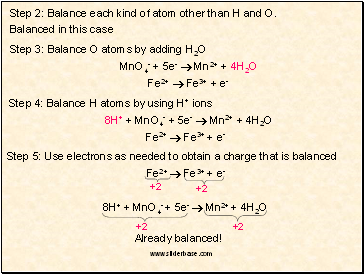Step 2: Balance each kind of atom other than H and O.

Balanced in this case

Step 3: Balance O atoms by adding H2O

MnO4- + 5e-  Mn2+ + 4H2O

Fe2+  Fe3+ + e-

Step 4: Balance H atoms by using H+ ions

8H+ + MnO4- + 5e-  Mn2+ + 4H2O

Fe2+  Fe3+ + e-

Step 5: Use electrons as needed to obtain a charge that is balanced

Fe2+  Fe3+ + e-

8H+ + MnO4- + 5e-  Mn2+ + 4H2O

+2

+2

+2

+2

Slide 20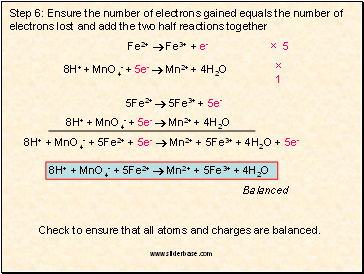Step 6: Ensure the number of electrons gained equals the number of electrons lost and add the two half reactions together

Fe2+  Fe3+ + e-

8H+ + MnO4- + 5e-  Mn2+ + 4H2O

× 5

× 1

5Fe2+  5Fe3+ + 5e-

8H+ + MnO4- + 5e-  Mn2+ + 4H2O

8H+ + MnO4- + 5Fe2+ + 5e-  Mn2+ + 5Fe3+ + 4H2O + 5e-

8H+ + MnO4- + 5Fe2+  Mn2+ + 5Fe3+ + 4H2O

Check to ensure that all atoms and charges are balanced.

Balanced

Slide 21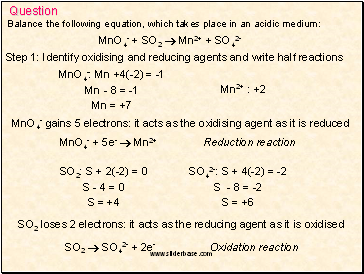Balance the following equation, which takes place in an acidic medium:

Question

MnO4- + SO2  Mn2+ + SO42-

Step 1: Identify oxidising and reducing agents and write half reactions

MnO4-: Mn +4(-2) = -1

Mn - 8 = -1

Mn = +7

Mn2+ : +2

MnO4- gains 5 electrons: it acts as the oxidising agent as it is reduced

MnO4- + 5e-  Mn2+ Reduction reaction

SO2: S + 2(-2) = 0

S - 4 = 0

S = +4

SO42-: S + 4(-2) = -2

S - 8 = -2

S = +6

SO2 loses 2 electrons: it acts as the reducing agent as it is oxidised

SO2  SO42- + 2e- Oxidation reaction

Slide 22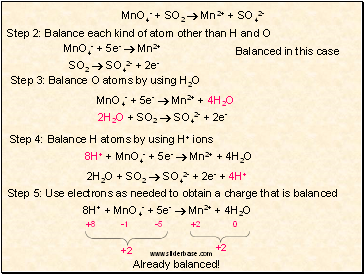Step 2: Balance each kind of atom other than H and O

Balanced in this case

Step 3: Balance O atoms by using H2O

MnO4- + 5e-  Mn2+ + 4H2O

2H2O + SO2  SO42- + 2e-

Step 4: Balance H atoms by using H+ ions

8H+ + MnO4- + 5e-  Mn2+ + 4H2O

2H2O + SO2  SO42- + 2e- + 4H+

Step 5: Use electrons as needed to obtain a charge that is balanced

8H+ + MnO4- + 5e-  Mn2+ + 4H2O

+2

+2

MnO4- + SO2  Mn2+ + SO42-

MnO4- + 5e-  Mn2+

SO2  SO42- + 2e-

-5

+2

0

-1

+8

Slide 23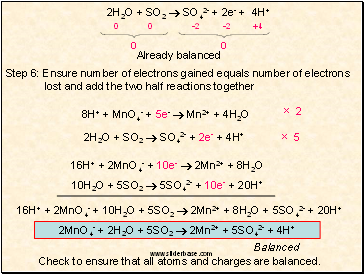2H2O + SO2  SO42- + 2e- + 4H+

0

0

Step 6: Ensure number of electrons gained equals number of electrons lost and add the two half reactions together

Go to page:
1  2  3  4  5  6  7  8